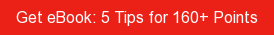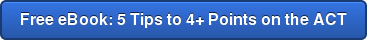# SAT / ACT Prep Online Guides and TipsStudents in AP Chemistry need to memorize a lot of information, but we have some good news! During the AP exam, you'll have access to a formula sheet that includes a periodic table as well as useful equations. However, you'll need to have a good sense of what's on the AP Chem formula sheet and how to use it in order to make the most of it during the test.

In this guide, we explain everything you need to know about the AP Chemistry reference table. We go over what the formula sheet looks like, what it includes, what important information it doesn't contain but that you should know, and how you can get the most out of it.

## What's on the AP Chem Formula Sheet?

The AP Chemistry reference sheet is actually three pages of information. It will be at the beginning of both the multiple-choice and free-response sections of the test, so you'll have access to it for the entire AP Chemistry exam. This means you don't need to memorize any of the information the formula sheet contains.

So what's on this AP Chem reference sheet? Check it out here on pages 2-4, as well as the screenshots below (click on each image to enlarge).

The AP Chem equation sheet covers six main topics.

• Periodic table of elements
• Atomic structure
• Equilibrium
• Kinetics
• Gases, liquids, and solutions
• Thermodynamics/electrochemistryPeriodic Table of Elements

The AP Chem equation sheet includes a complete periodic table. For each element, it includes the element name, symbol, atomic number, and atomic mass.

Atomic Structure

• 2 equations
• Values for:
• Planck's constant
• Speed of light
• Electron charge

Equilibrium

• 9 equations

Kinetics

• 3 equations

Gases, Liquids, and Solutions

• 9 equations
• Values for:
• Gas constant (3)
• 1 atm
• STP
• Ideal gas at STP

Thermodynamics/Electrochemistry

• 9 equations
• Values for:## What Isn't on the AP Chem Formula Sheet That You Need to Know for the Exam?

While the AP Chemistry reference table does a good job of covering the majority of equations you need to know for the exam, there is some information it's missing. That's what we cover in this section. Be sure to know these equations for the exam.

### Rate Law

One of the laws you learn in AP Chemistry is that, at a constant temperature, the rate of a chemical reaction depends only on the concentrations of the substances that influence the rate. These substances are typically reactants, but can also include products and catalysts.

The rate law formula is: rate=k[A]a[B]b

[A] and [B] represent molar concentrations of reactants
k is the rate constant (which varies depending on the reaction and the temperature)
a and b are typically positive integers that must be found experimentally

Rate law is used to estimate the relationship between the rate of a reaction and the concentrations of reactants. You may see it on questions related to kinetics.

### Coulomb's Law

Coulomb's Law states that magnitude of electric force between the charges q1 and q2 is directly proportional to the magnitude of the charges and inversely proportional to the square of the distance between them. You may see it on electrochemistry questions.

F=k(q1q2/r)

F= electric force
k= Coulomb constant
q1 and q2= charges
r= distance of separation

### Important Percentages

You might need to use these equations on any portion of the test. They determine how accurate estimates are compared to actual results.

%error = ((actual value - theoretical value)/(theoretical value))*100

%yield = (actual yield/theoretical yield)*100

## 4 Tips for Getting the Most Out of the AP Chem Equation Sheet

The AP Chemistry formula sheet can be extremely helpful during the exam, but only if you know how to use it properly. Here are four tips for getting the most out of it.

### #1: Know How to Use Every Equation on the AP Chem Formula Sheet

The AP Chem reference sheet contains 32 equations, and you should know how each of them works before test day. The College Board isn't one for including extraneous information on formula sheets, so if a formula is included on the sheet, that means there's a solid chance you'll need to use it on the exam. You don't want to waste your exam trying to learn how to use, say Planck's equation, and hoping you got it right!

Well before exam day, be sure to go through every formula on the sheet and make sure you understand it and know how to use it. The keys included in the formula sheet are also helpful for figuring out what different variables represent in the equations, so don't neglect them either.

### #2: Use Your Calculator Wisely

You're allowed a graphing calculator on the free-response portion of the exam, but not the multiple-choice section. You can make good use of your calculator by programming constants and formulas into it so you can solve equations more easily. However, don't think this means you get out of understanding the actual formulas themselves. You still need to know when and how to use each equation on the AP Chemistry reference table, not to mention you won't have your calculator for multiple-choice questions. So know when your calculator is useful, but don't over-rely on it.

### #3: Take Practice Tests Using the Formula Sheet

Answering practice questions and taking practice tests using the AP Chem formula sheet is key to doing well on the exam. You should take several AP Chemistry practice tests before the actual AP exam. For each of them, use the official formula sheet. Your teacher will also likely give you a copy of the AP Chem formula sheet for your in-class exams, so you can get some practice in there too.

If you need help finding practice tests, check out our guide specifically on where to find the best AP Chemistry practice questions and tests.

### #4: Become an Expert on the Periodic Table

If there's one guarantee for the AP Chemistry exam, it's that you'll need to look at the included periodic table during the test. The periodic table included in the AP Chemistry equation sheet has all the information you'll need to answer questions about it. However, that doesn't mean you can ignore it until it comes time to take the test. It's very important to be familiar with the periodic table throughout the year. You should know what each value in the table represents, how to use group numbers to determine the number of bonds and valence electrons an element can form, and what the key periodic trends are. Spend the most time on groups 1-8A, as you'll be tested on these elements most often.## What's Next?

How are the AP Physics classes and exams different? Learn the difference between Physics 1, Physics 2, and Physics C in our guide to AP Physics classes.

How many AP courses total should you take? What if you want to get into an Ivy League school? Read our advice on AP course load here.

Also studying for the ACT/SAT? Come up with a target ACT or SAT score based on your top schools.

Want to improve your SAT score by 160 points or your ACT score by 4 points? We've written a guide for each test about the top 5 strategies you must be using to have a shot at improving your score. Download it for free now: